# 利用 MQL5 和 MQL4 实现的选择和导航工具: 把数据添加到图表中

31 五月 2019, 08:580
4 683

### 隐藏今天的趋势与昨天不同的交易品种

```/*

symname - 使用的交易品种名称
*/
bool check_no_trend(string symname){
MqlRates rates2[];
ArraySetAsSeries(rates2, true);
if(CopyRates(symname, PERIOD_D1, 0, 2, rates2)==2){
if( rates2.close>rates2.open && rates2.close>rates2.open ){
return false;
}
if( rates2.close<rates2.open && rates2.close<rates2.open ){
return false;
}
}
return true;
}
```

### 显示开盘时间

```/*

currChart - 图表 id
day - 日期编号 (0 - 当前)
*/
void showNdays(long currChart, int day){
MqlRates rates[];
ArraySetAsSeries(rates, true);
if(CopyRates(ChartSymbol(currChart), PERIOD_D1, day, 1, rates)>0){
ObjectCreate(currChart, exprefix+"_Nday", OBJ_VLINE, 0, rates.time, 0);
ObjectSetInteger(currChart,exprefix+"_Nday",OBJPROP_STYLE,STYLE_DOT);
ObjectSetInteger(currChart,exprefix+"_Nday",OBJPROP_RAY,true);
ObjectSetInteger(currChart,exprefix+"_Nday",OBJPROP_SELECTABLE,false);
ObjectSetInteger(currChart,exprefix+"_Nday",OBJPROP_BACK,true);
}
}
```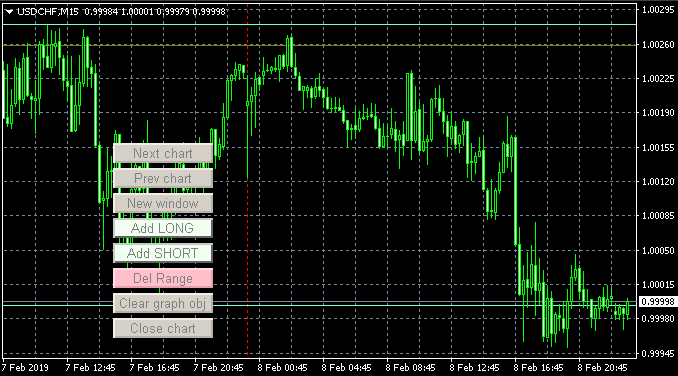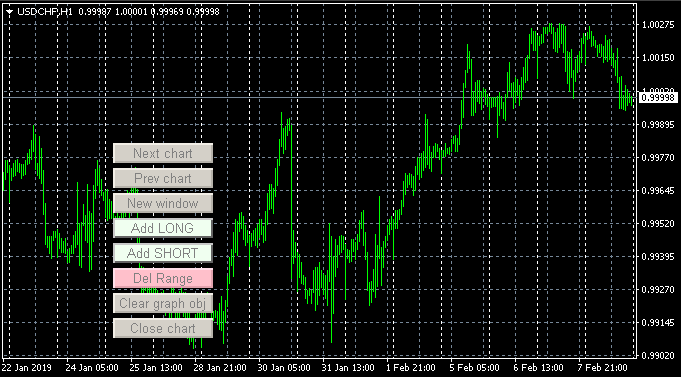### 显示昨天和前天的最低价和最高价

```/*

currChart - 图表 ID
day - 显示日期的索引
*/
void showHOBeforeDay(long currChart, int day=1){
MqlRates rates[];
ArraySetAsSeries(rates, true);

color curColor=clrAquamarine;
switch(day){
case 2:
curColor=clrOlive;
break;
}

if(CopyRates(ChartSymbol(currChart), PERIOD_D1, day, 1, rates)>0){
ObjectCreate(currChart, exprefix+"_beforeday_high_line"+(string) day, OBJ_HLINE, 0, 0, rates.high);
ObjectSetInteger(currChart,exprefix+"_beforeday_high_line"+(string) day,OBJPROP_COLOR,curColor);
ObjectSetInteger(currChart,exprefix+"_beforeday_high_line"+(string) day,OBJPROP_SELECTABLE,false);
ObjectSetInteger(currChart,exprefix+"_beforeday_high_line"+(string) day,OBJPROP_BACK,true);

ObjectCreate(currChart, exprefix+"_beforeday_low_line"+(string) day, OBJ_HLINE, 0, 0, rates.low);
ObjectSetInteger(currChart,exprefix+"_beforeday_low_line"+(string) day,OBJPROP_COLOR,curColor);
ObjectSetInteger(currChart,exprefix+"_beforeday_low_line"+(string) day,OBJPROP_SELECTABLE,false);
ObjectSetInteger(currChart,exprefix+"_beforeday_low_line"+(string) day,OBJPROP_BACK,true);
}
}
```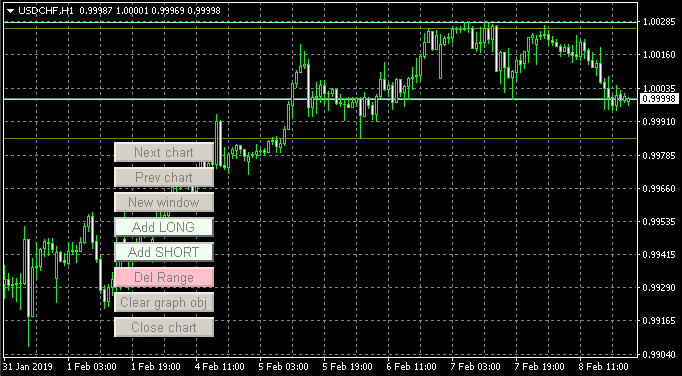### 显示最近的整数价格

.25 和 .75. 级别也可能被认为是整数的，但它们不太可靠，因此我们不会跟踪它们，以免使图表混乱。

```/*

currChart - 图表 id
*/
void showRoundPrice(long currChart){
string name=ChartSymbol(currChart);
int tmpPrice;

MqlRates rates[];
ArraySetAsSeries(rates, true);
if(CopyRates(name, PERIOD_D1, 0, 1, rates)==1){
switch((int) SymbolInfoInteger(name, SYMBOL_DIGITS)){
case 0:
break;
case 2:
case 3:
case 5:
tmpPrice=(int) rates.close;

ObjectCreate(currChart, exprefix+"_round_line_00_0", OBJ_HLINE, 0, 0, (double) tmpPrice-1 );
ObjectSetInteger(currChart,exprefix+"_round_line_00_0",OBJPROP_STYLE, STYLE_DASH);
ObjectSetInteger(currChart,exprefix+"_round_line_00_0",OBJPROP_SELECTABLE,false);
ObjectSetInteger(currChart,exprefix+"_round_line_00_0",OBJPROP_BACK,true);

ObjectCreate(currChart, exprefix+"_round_line_05_0", OBJ_HLINE, 0, 0, (double) tmpPrice-0.5 );
ObjectSetInteger(currChart,exprefix+"_round_line_05_0",OBJPROP_STYLE, STYLE_DASH);
ObjectSetInteger(currChart,exprefix+"_round_line_05_0",OBJPROP_SELECTABLE,false);
ObjectSetInteger(currChart,exprefix+"_round_line_05_0",OBJPROP_BACK,true);

ObjectCreate(currChart, exprefix+"_round_line_00_1", OBJ_HLINE, 0, 0, (double) tmpPrice );
ObjectSetInteger(currChart,exprefix+"_round_line_00_1",OBJPROP_STYLE, STYLE_DASH);
ObjectSetInteger(currChart,exprefix+"_round_line_00_1",OBJPROP_SELECTABLE,false);
ObjectSetInteger(currChart,exprefix+"_round_line_00_1",OBJPROP_BACK,true);

ObjectCreate(currChart, exprefix+"_round_line_05_1", OBJ_HLINE, 0, 0, (double) tmpPrice+0.5 );
ObjectSetInteger(currChart,exprefix+"_round_line_05_1",OBJPROP_STYLE, STYLE_DASH);
ObjectSetInteger(currChart,exprefix+"_round_line_05_1",OBJPROP_SELECTABLE,false);
ObjectSetInteger(currChart,exprefix+"_round_line_05_1",OBJPROP_BACK,true);

ObjectCreate(currChart, exprefix+"_round_line_00_2", OBJ_HLINE, 0, 0, (double) tmpPrice+1 );
ObjectSetInteger(currChart,exprefix+"_round_line_00_2",OBJPROP_STYLE, STYLE_DASH);
ObjectSetInteger(currChart,exprefix+"_round_line_00_2",OBJPROP_SELECTABLE,false);
ObjectSetInteger(currChart,exprefix+"_round_line_00_2",OBJPROP_BACK,true);

ObjectCreate(currChart, exprefix+"_round_line_05_2", OBJ_HLINE, 0, 0, (double) tmpPrice+1.5 );
ObjectSetInteger(currChart,exprefix+"_round_line_05_2",OBJPROP_STYLE, STYLE_DASH);
ObjectSetInteger(currChart,exprefix+"_round_line_05_2",OBJPROP_SELECTABLE,false);
ObjectSetInteger(currChart,exprefix+"_round_line_05_2",OBJPROP_BACK,true);

break;
}
}
}

```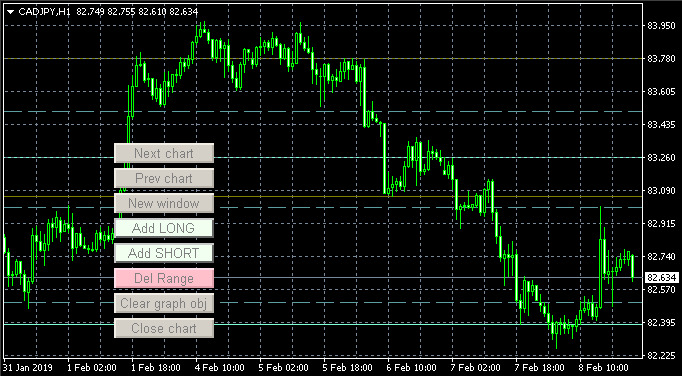### 显示小时结束

```/*

currChart - 图表 id
*/
void showCloseHour(long currChart){
MqlDateTime tmpTime;
int tmpOffset=0;
MqlRates rates[];
ArraySetAsSeries(rates, true);
if(CopyRates(ChartSymbol(currChart), PERIOD_M30, 0, 9, rates)>0){
tmpOffset=0;
TimeToStruct(rates[tmpOffset].time, tmpTime);
if(tmpTime.min!=0){
tmpOffset++;
}
ObjectCreate(currChart, exprefix+"_hour_0", OBJ_VLINE, 0, rates[tmpOffset].time, 0);
ObjectSetInteger(currChart,exprefix+"_hour_0",OBJPROP_SELECTABLE,false);
ObjectSetInteger(currChart,exprefix+"_hour_0",OBJPROP_STYLE,STYLE_DOT);
ObjectSetInteger(currChart,exprefix+"_hour_0",OBJPROP_RAY,true);
ObjectSetInteger(currChart,exprefix+"_hour_0",OBJPROP_COLOR,clrYellow);
ObjectSetInteger(currChart,exprefix+"_hour_0",OBJPROP_BACK,true);

tmpOffset++;
TimeToStruct(rates[tmpOffset].time, tmpTime);
if(tmpTime.min!=0){
tmpOffset++;
}
ObjectCreate(currChart, exprefix+"_hour_1", OBJ_VLINE, 0, rates[tmpOffset].time, 0);
ObjectSetInteger(currChart,exprefix+"_hour_1",OBJPROP_SELECTABLE,false);
ObjectSetInteger(currChart,exprefix+"_hour_1",OBJPROP_STYLE,STYLE_DOT);
ObjectSetInteger(currChart,exprefix+"_hour_1",OBJPROP_RAY,true);
ObjectSetInteger(currChart,exprefix+"_hour_1",OBJPROP_COLOR,clrYellow);
ObjectSetInteger(currChart,exprefix+"_hour_1",OBJPROP_BACK,true);

tmpOffset++;
TimeToStruct(rates[tmpOffset].time, tmpTime);
if(tmpTime.min!=0){
tmpOffset++;
}
ObjectCreate(currChart, exprefix+"_hour_2", OBJ_VLINE, 0, rates[tmpOffset].time, 0);
ObjectSetInteger(currChart,exprefix+"_hour_2",OBJPROP_SELECTABLE,false);
ObjectSetInteger(currChart,exprefix+"_hour_2",OBJPROP_STYLE,STYLE_DOT);
ObjectSetInteger(currChart,exprefix+"_hour_2",OBJPROP_RAY,true);
ObjectSetInteger(currChart,exprefix+"_hour_2",OBJPROP_COLOR,clrYellow);
ObjectSetInteger(currChart,exprefix+"_hour_2",OBJPROP_BACK,true);

tmpOffset++;
TimeToStruct(rates[tmpOffset].time, tmpTime);
if(tmpTime.min!=0){
tmpOffset++;
}
ObjectCreate(currChart, exprefix+"_hour_3", OBJ_VLINE, 0, rates[tmpOffset].time, 0);
ObjectSetInteger(currChart,exprefix+"_hour_3",OBJPROP_SELECTABLE,false);
ObjectSetInteger(currChart,exprefix+"_hour_3",OBJPROP_STYLE,STYLE_DOT);
ObjectSetInteger(currChart,exprefix+"_hour_3",OBJPROP_RAY,true);
ObjectSetInteger(currChart,exprefix+"_hour_3",OBJPROP_COLOR,clrYellow);
ObjectSetInteger(currChart,exprefix+"_hour_3",OBJPROP_BACK,true);

}
}
```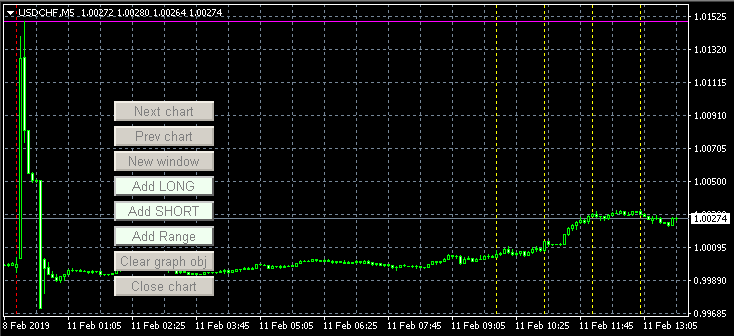### 显示一年的最高和最低价格

Input: 显示一年的最高/最低价

```/*

currChart - 图表 id.
*/
void showMaxPrice(long currChart){
MqlRates rates[];
ArraySetAsSeries(rates, true);
if(CopyRates(ChartSymbol(currChart), PERIOD_MN1, 0, 12, rates)==12){
double maxPrice=0;
double minPrice=0;

for(int j=0; j<12; j++){
if( maxPrice==0 || maxPrice<rates[j].high ){
maxPrice=rates[j].high;
}
if( minPrice==0 || minPrice>rates[j].low ){
minPrice=rates[j].low;
}
}

ObjectCreate(currChart, exprefix+"_maxprice_line", OBJ_HLINE, 0, 0, maxPrice);
ObjectSetInteger(currChart,exprefix+"_maxprice_line",OBJPROP_COLOR,clrMagenta);
ObjectSetInteger(currChart,exprefix+"_maxprice_line",OBJPROP_SELECTABLE,false);
ObjectSetInteger(currChart,exprefix+"_maxprice_line",OBJPROP_BACK,true);

ObjectCreate(currChart, exprefix+"_minprice_line", OBJ_HLINE, 0, 0, minPrice);
ObjectSetInteger(currChart,exprefix+"_minprice_line",OBJPROP_COLOR,clrMagenta);
ObjectSetInteger(currChart,exprefix+"_minprice_line",OBJPROP_SELECTABLE,false);
ObjectSetInteger(currChart,exprefix+"_minprice_line",OBJPROP_BACK,true);

}
}
```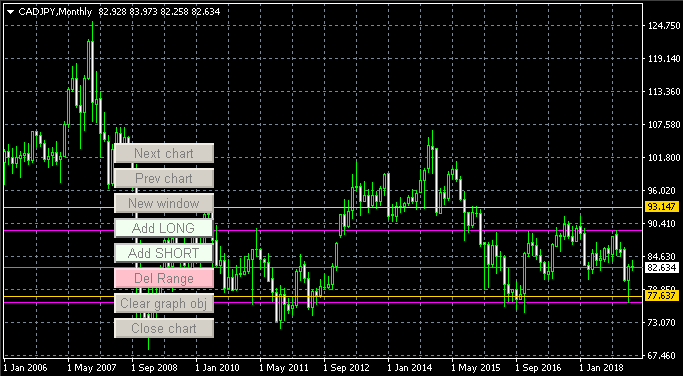### 当打开图表时显示点差

```/*

symname - 交易品种名称
*/
}

```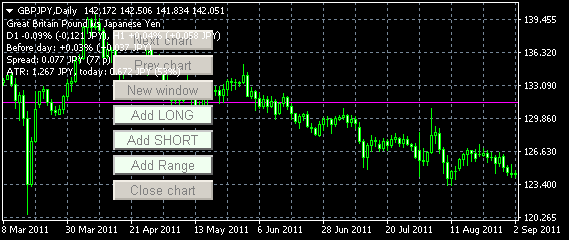### 根据名称在图表上应用模板

```      ChartApplyTemplate(curChartID[ArraySize(curChartID)-1], allApplyTemplate+".tpl");

```

### 显示 Gerchik's ATR

ATR是交易品种在一定时间内的平均日移动量，是确定交易品种作用范围的重要参数。它有多种不同用途，

Gerchik's ATR 与传统的ATR不同的是，只考虑了异常的柱。异常柱是指比平均柱大2倍或小0.3倍的柱。

ATR通常计算5或3天。

```/*

symname - 交易品种名称
*/
string getmeatr_symbol(string symname){
string msg="";
MqlRates rates[];
ArraySetAsSeries(rates, true);
double atr=0;
double pre_atr=0;
int curDigits=(int) SymbolInfoInteger(symname, SYMBOL_DIGITS);
string currencyS=SymbolInfoString(symname, SYMBOL_CURRENCY_PROFIT);
int count=0;
int copied=CopyRates(symname, PERIOD_D1, 0, 8, rates);
if(copied>1){
for(int j=1; j<copied; j++){
pre_atr+=rates[j].high-rates[j].low;
}
pre_atr/=(copied-1);
}
if(pre_atr>0){
for(int j=1; j<copied; j++){
if( rates[j].high-rates[j].low > pre_atr*2 ) continue;
if( rates[j].high-rates[j].low < pre_atr*0.3 ) continue;

if( ++count > 5 ){
break;
}

atr+=rates[j].high-rates[j].low;
}
if( count > 5 ){
count=5;
}
atr= NormalizeDouble(atr/count, curDigits );
}
if(atr>0){
StringAdd(msg, "ATR: "+(string) DoubleToString(atr, curDigits)+" "+currencyS+", today: "+DoubleToString(rates.high-rates.low, curDigits)+" "+currencyS+" ("+(string) (int) (((rates.high-rates.low)/atr)*100) +"%)");
}

return msg;
}
```

### 导向交易品种的显示方向

• 美国主要指数，至少是道琼斯或SP500；
• 其余国家的一个指数：中国、欧洲、日本；
• 各细分市场的大型股公司：摩根大通银行业、苹果科技、埃克森美孚能源、IBM等。

```/*

symname - 交易品种名称
show - 字符串中是否应显示交易品种名称标签
*/
string getmeinfo_symbol(string symname, bool show=true){
MqlRates rates2[];
ArraySetAsSeries(rates2, true);
string msg="";

if(CopyRates(symname, PERIOD_D1, 0, 1, rates2)>0){
if(show){
}
if( rates2.close > rates2.open ){
StringAdd(msg, "+"+DoubleToString(((rates2.close-rates2.open)/rates2.close)*100, 2) +"% (+"+DoubleToString(rates2.close-rates2.open, (int) SymbolInfoInteger(symname, SYMBOL_DIGITS))+" "+SymbolInfoString(symname, SYMBOL_CURRENCY_PROFIT)+")");
}else{
if( rates2.close < rates2.open ){
StringAdd(msg, "-"+DoubleToString(((rates2.open-rates2.close)/rates2.close)*100, 2) +"% (-"+DoubleToString(rates2.open-rates2.close, (int) SymbolInfoInteger(symname, SYMBOL_DIGITS))+" "+SymbolInfoString(symname, SYMBOL_CURRENCY_PROFIT)+")");
}else{
}
}
}
if(CopyRates(symname, PERIOD_H1, 0, 1, rates2)>0){
if( rates2.close > rates2.open ){
StringAdd(msg, "+"+DoubleToString(((rates2.close-rates2.open)/rates2.close)*100, 2)+"% (+"+DoubleToString(rates2.close-rates2.open, (int) SymbolInfoInteger(symname, SYMBOL_DIGITS))+" "+SymbolInfoString(symname, SYMBOL_CURRENCY_PROFIT)+")");
}else{
if( rates2.close < rates2.open ){
StringAdd(msg, "-"+DoubleToString(((rates2.open-rates2.close)/rates2.close)*100, 2)+"% (-"+DoubleToString(rates2.open-rates2.close, (int) SymbolInfoInteger(symname, SYMBOL_DIGITS))+" "+SymbolInfoString(symname, SYMBOL_CURRENCY_PROFIT)+")");
}else{
}
}
}

return msg;
}
```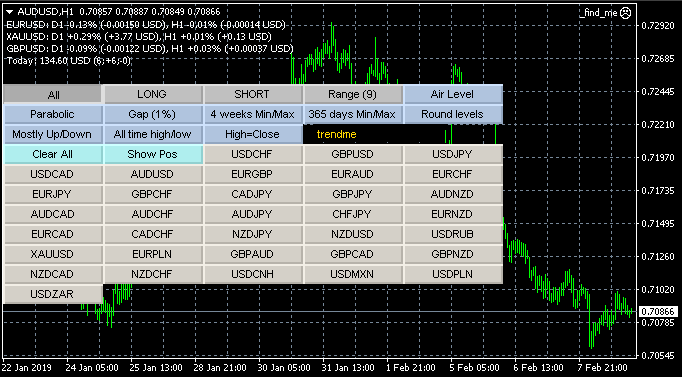### 自动更新实用程序选项卡

```   // 指定秒数后更新选项卡的内容
if( autoUpdateAfter>0 && TimeCurrent()-lastUpd >= autoUpdateAfter ){
start_symbols();
lastUpd=TimeCurrent();
}

```

### 显示股票的财务数据

```void printTicker(string ticker, string name){
bool isYes=false;
int filehandle;

// 从分时器中删除多余的后缀和前缀
// 并将其转换为小写
StringToLower(ticker);
StringReplace(ticker, "#", "");
StringReplace(ticker, ".us", "");
StringReplace(ticker, ".eu", "");

// 将公司名称转换为小写
StringToLower(name);

ResetLastError();
// 如果“finder”文件夹中包含公司的数据，则显示该文件夹。
if( FileIsExist("finder\\"+ticker+".dat") ){
isYes=true;
if(filehandle!=INVALID_HANDLE){
int str_size;
string str;
Print("----------------");
while(!FileIsEnding(filehandle)){
Print(str);
}
FileClose(filehandle);
}
}else if( FileIsExist("finder\\"+name+".dat") ){
isYes=true;
if(filehandle!=INVALID_HANDLE){
int str_size;
string str;
Print("----------------");
while(!FileIsEnding(filehandle)){
Print(str);
}
FileClose(filehandle);
}
}

// 否则，通知未找到数据
if(!isYes){
Print("没有交易品种数据");
}
}
```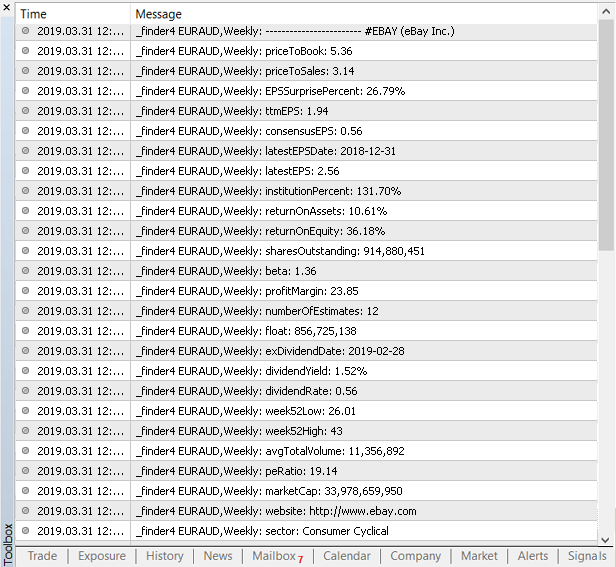### 显示今天的利润

```#ifdef __MQL5__
if(orders_total==PositionsTotal()){
return;
}
if(orders_total>PositionsTotal()){
needHistory=true;
}
orders_total=(ushort) PositionsTotal();
}
#endif
/*

*/
string getmetoday_profit(){
string msg="";
double curProfit=0;

MqlDateTime curD;
TimeCurrent(curD);
curD.hour=0;
curD.min=0;
curD.sec=0;

#ifdef __MQL5__
if( needHistory ){
HistorySelect(StructToTime(curD),TimeCurrent());
}
uint totalHistory=HistoryDealsTotal();
double currHistory=0;
ulong ticket_history;
for(uint j=0;j<totalHistory;j++){
if((ticket_history=HistoryDealGetTicket(j))>0 ){
double profitHistory=HistoryDealGetDouble(ticket_history,DEAL_PROFIT);
profitHistory+=HistoryDealGetDouble(ticket_history,DEAL_COMMISSION);
profitHistory+=HistoryDealGetDouble(ticket_history,DEAL_SWAP);
if(profitHistory!=0){
currHistory+=profitHistory;
}
}
}

#else
int cntMyPos=OrdersHistoryTotal();
if(cntMyPos>0){
for(int ti=cntMyPos-1; ti>=0; ti--){
if(OrderSelect(ti,SELECT_BY_POS,MODE_HISTORY)==false) continue;

if( OrderCloseTime()<StructToTime(curD) ){ continue; }

double tmpProfit=OrderProfit();
tmpProfit+=OrderSwap();
tmpProfit+=OrderCommission();

if(tmpProfit>0){
}else if(tmpProfit<0){
}
curProfit+=tmpProfit;
}
}
#endif

}

return msg;
}
```### 结论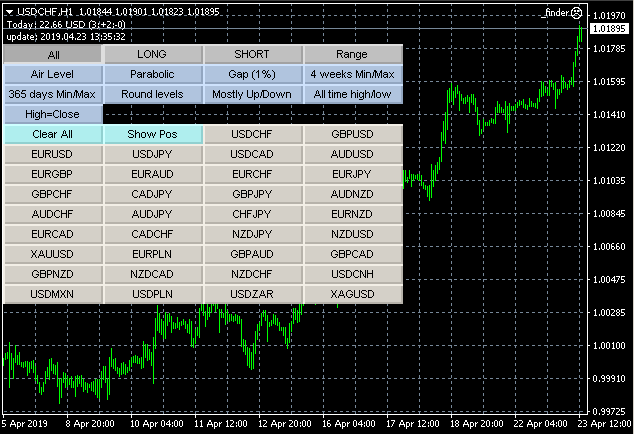All选项卡中显示的交易品种列表取决于过滤设置组: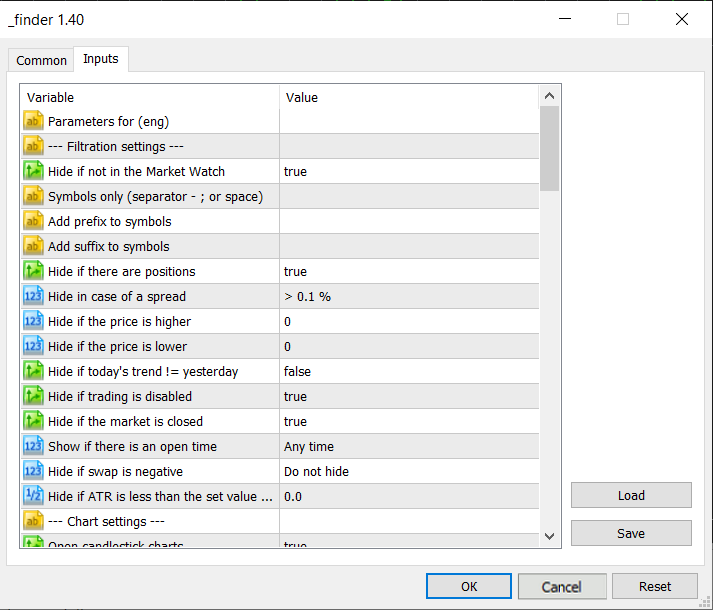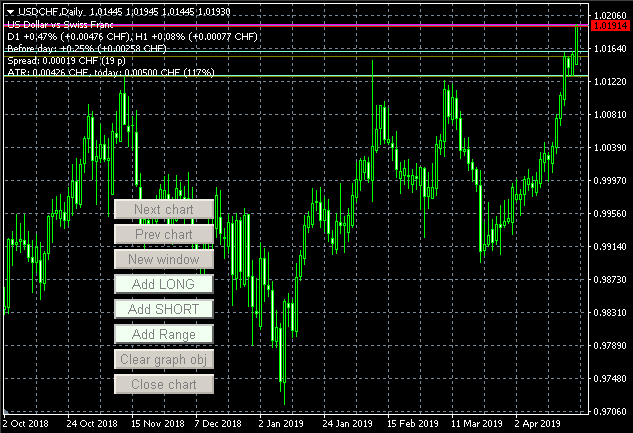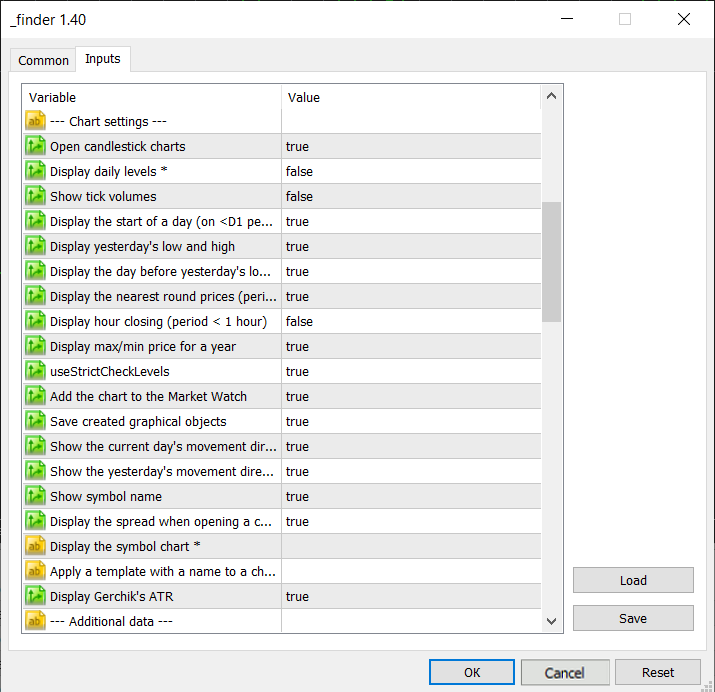All选项卡的时间框架是在附加数据中设置的。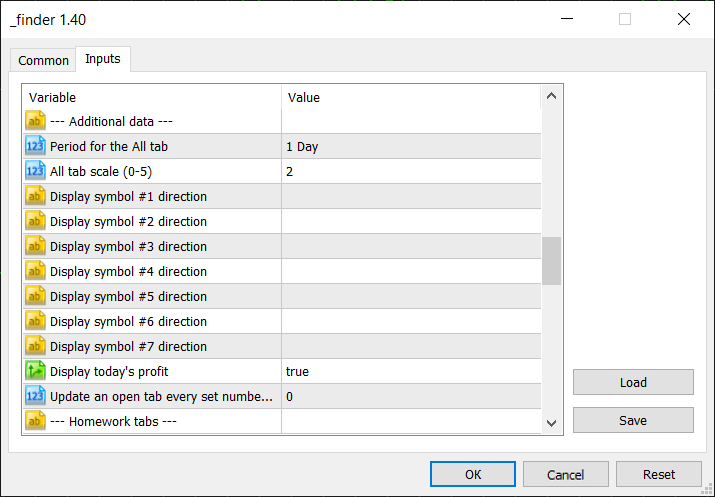All选项卡上所有的交易品种按钮，默认使用 D1 时段。让我们把它改为 M15: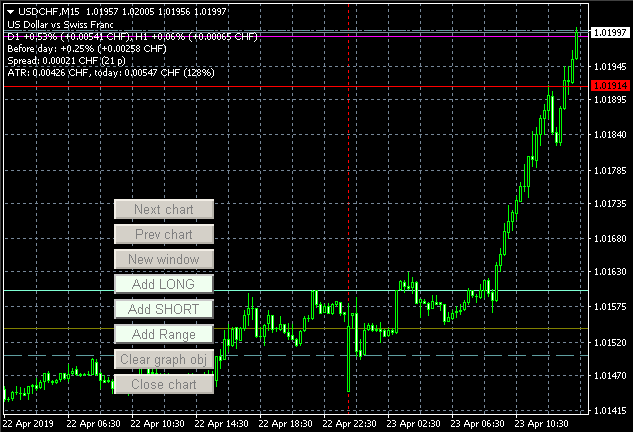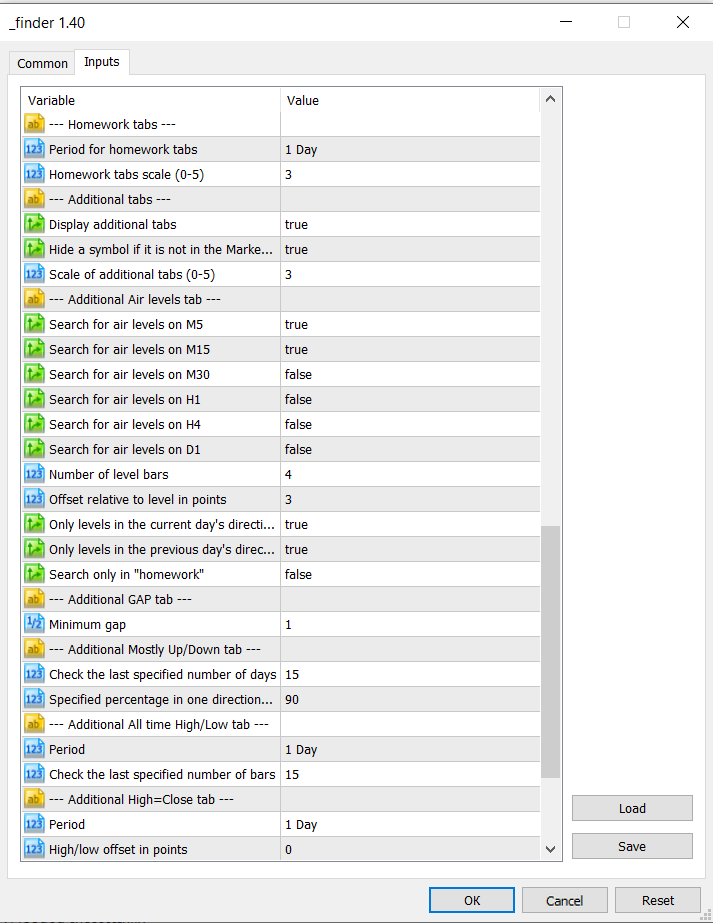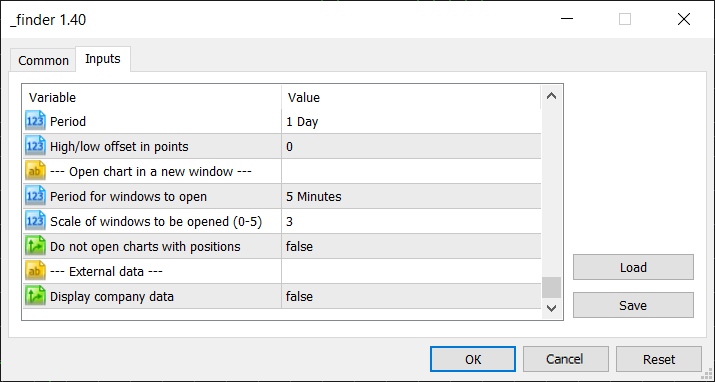finder.zip (8456.89 KB)
_finder.ex5 (354.46 KB)
_finder.mq5 (258.68 KB)
_finder.ex4 (230.82 KB)
_finder.mq4 (258.68 KB)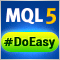轻松快捷开发 MetaTrader 程序的函数库(第四部分)：交易事件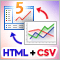如何基于HTML和CSV报表可视化多币种交易历史轻松快捷开发 MetaTrader 程序的函数库(第五部分)：交易事件集合类，向程序发送事件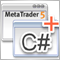基于 .Net 框架和 C# 开发图形界面(第二部分)：另外的图形元素